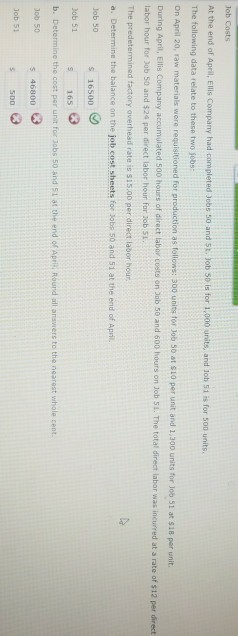# Question & Answer: At the end of April, Ellis Company had completed Jobs 50 and 51. Job 50 is for 1,000 units, and job 51 is for 500 units. The following dat…..Job cost.

Don't use plagiarized sources. Get Your Custom Essay on
Question & Answer: At the end of April, Ellis Company had completed Jobs 50 and 51. Job 50 is for 1,000 units, and job 51 is for 500 units. The following dat…..
GET AN ESSAY WRITTEN FOR YOU FROM AS LOW AS \$13/PAGE

At the end of April, Ellis Company had completed Jobs 50 and 51. Job 50 is for 1,000 units, and job 51 is for 500 units. The following data relate to these two jobs: On April 20, raw materials were for production as follow: 300 units for Job 50 at \$10 per units and 1,300 units for Jon 51 at \$18 per unit. During April, Ellis Company accumulated 500 hours of direct labor costs on Job 50 and 600 hours on Job 51. The total direct was incurred at a rate of \$12 per direct labor hour for Job 50 and \$24 per direct labor hour for Job \$1. The predetermined factory overhead rate is \$15.00 per direct labor hour. a. Determine the balance on the job cost sheets for Jobs 50 and 51 at the end of April. Job 50 Job 51 b. Determine the cost per unit for Jobs \$0 and \$1 at the end of April. Around all answers to the nearest whole cent. Job 50 Job 51

a.

The balance on the job cost sheet for Jobs 50 and 51 at the end of April will be determined as follows:

 Job 50 Job 51 Direct materials cost 300×10=3000 1300×18=23400 Direct labor cost 500×12=6000 500×24=12000 Factory overhead 500×15=7500 500×15=7500 Balance on the job cost sheet 3000+6000+7500=16500 23400+12000+7500=42900

b.

Cost per unit for Jobs 50 and 51 will be determined as follows:

Cost per unit for Job 50 = Total cost / Number of units = \$16,500/1,000 units = \$16.50

Cost per unit for Job 51 = Total cost / Number of units = \$42,900/500 units = \$85.80